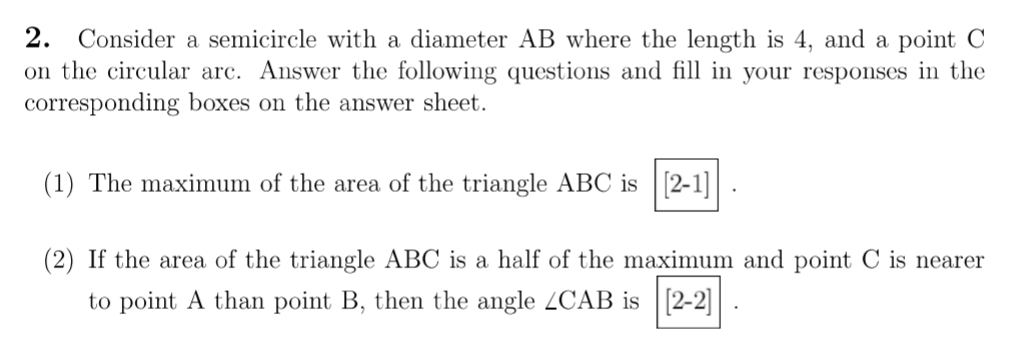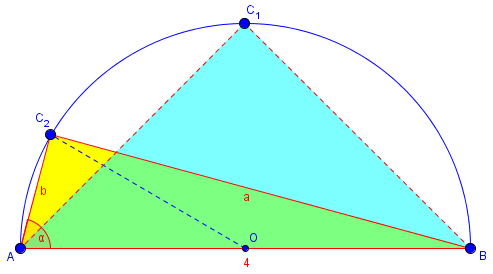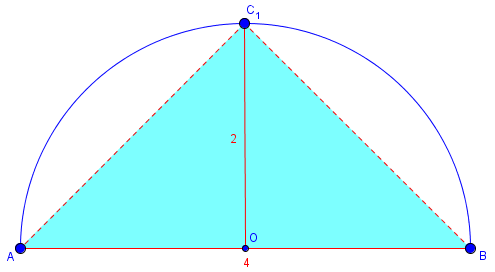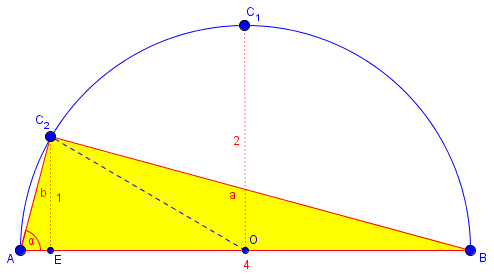# A Triangle in a Semicircle

#### (A new question of the week)

Like many questions we get, this one can be solved in many ways. We like to guide a student to whatever solution will fit what they have learned; along the way, we may find various additional methods, and side trips into other topics of interest.

## A two-part problem

This comes from Kurisada, who has sent us many good problems from his (her?) review of many areas of math, at the end of last December:

Hello Dr. Math

May I know the way of the problem 2(2) below?Incidentally, I find it interesting how the language used in English-language problems from around the world can vary; this helps me not let “errors” in students’ English bother me. For what it’s worth, here is how I would have worded the problem:

Consider a semicircle with diameter AB = 4, with point C on the circular arc.

(1) The maximum possible area of triangle ABC is ___.

(2) If the area of triangle ABC is half of the maximum, and point C is nearer to point A than point B, then angle CAB is ___.

Here is a picture of what the question is asking:Point $$C_1$$ is the position of C that yields the greatest possible area for triangle ABC, which we are first asked for (part 1); the position shown for $$C_2$$ yields half of that area.We want to find angle α (part 2). There is a very quick way to find each of these; but our goal is to help the student discover that he can solve a problem himself, not just to give the easy answer.

As we commonly do for questions like this, I asked for Kurisada’s ideas, while giving hints for possible methods:

There are many ways you might work on this problem; that is one of the reasons we ask you to show your own work. Do you want to use coordinate geometry, perhaps, or trigonometry, or calculus? Some or all of these may be helpful, in addition to ordinary geometry; but you have given no hint as to which of them you know.

You imply that you have the answer to part (1). What is your answer? How did you get it? That will play a role in the next part.

The second part probably requires trigonometry. Do you know whether an exact or approximate decimal answer is required?

From part (1) you can find the height of this triangle ABC. Again, there are several ways I can see to find required parts of this triangle in order to determine the angle. Please make a start, so I can see what methods you are comfortable with, and avoid suggesting ways that might be too hard for you; and also tell me what topics you are learning.

Because my focus was on getting information, I hadn’t given a lot of thought to the actual solution, which doesn’t really require trig. Doctor Rick supplemented my ideas:

Since you have labeled this as a geometry problem (and many geometry courses do not include trigonometry, though some do), let me point out that I see a way to solve it without trig. However, you do need to be familiar with the ratios of sides of some “special triangles”, so that you will recognize such a triangle from its ratios.

So, what do you know in this regard, and what have you done so far?

In fact, the solution to the problem (which asks only for an angle, not sides) is even easier than that. But all of the methods discussed will work, and the best way is always the one you see, not the one you will later wish you had seen!

## A trig solution for part 1

Kurisada responded with the information we wanted, showing work, and also the given answer (which we often need to know in case it is wrong):

1. For the first question, my answer is 4.
I did it by Triangle trigonometry ratio (4/sin 90 = x/sin 45).
Then I got x = 2√2.
Then I found the height = 2.
And area = 4.
2. In the second question, I’ve tried the same way but I couldn’t get the answer.
I also divided the triangle into two and get sin x = 0.5 {2√(2 – √3) + √(6 – 3√3)} and I stuck there.
I wonder if there is any special ratio in this triangle.

P.S.: the key answer shows that the answer is (5π)/12 or 75°.

I prefer either trigonometry or coordinate geometry to deepen my understanding in these topics.

My trigonometry and geometry ability is still basic to intermediate.

The first solution uses the Law of Sines applied to triangle $$AOC_1$$. The same result could be obtained by right angle trigonometry, or by merely recognizing OC as a radius.

The second, presumably using the Law of Sines again, needs further explanation.

## A geometrical solution for part 1

Thanks for letting us know that trigonometry is available for your solution.

You haven’t defined your x’s or stated where you put C in part 2, so it’s hard to follow your work.

For (1), I just see that the maximum possible height equals the radius, 2, so the area is bh/2 = 4*2/2 = 4. Your long way is valid.

For (2), I’m not sure exactly what you’ve done; but it was probably similar to my first attempt, which was not pretty.

The quick way (probably what Dr. Rick has in mind) is to draw the radius OC (O being the midpoint of AB) and think about triangles AOC and COB. Some simple trigonometry, or mere recognition of a special triangle as in (1), will tell you what ∠AOC is. No more trigonometry than that is needed.

I haven’t tried a coordinate geometry solution; that would probably be similar to my first attempt using trig.

My work for part (1):## More ideas for part 2

I just tried to draw the line OC.

I got AC = 4 cos x from:

AB / Sin C = AC / sin B

4 / sin 90 = AC / sin (90 – x)

And 4 = sin 2x from:

OC / sin A = AC / sin O        (the angle O is the same as 180 – 2x )

2 / sin x = 4 cos x / sin (180 – 2x)

And after several similar calculations, I still couldn’t find the answer .

Did I do something wrong?

“I just tried the draw the line OC

I got AC = 4 cos x from:

AB / Sin C = AC / sin B

4 / sin 90 = AC / sin (90 – x)”

You could just use the right-triangle definition of cosine, rather than apply the Law of Sines to right triangle ABC; but this is correct.

“And 4 = sin 2x from:

OC / sin x = AC / sin O        (the angle O is the same as 180 – 2x)

2 / sin x = 4 cos x / sin (180 – 2x) “

Here you applied the Law of Sines to isosceles triangle OAC. But this last equation is a tautology, since sin(180 – 2x) = sin(2x) = 2 sin(x)cos(x), so it doesn’t contribute anything. [In effect, you have proved the double-angle identity!]

I think you are relying on the Law of Sines too much. (“To a man with a hammer, everything is a nail!”) I would just use right-triangle definitions of trig functions, and geometry. Focus not on what tools you have, but on what you know about the problem.

You haven’t used the essential fact about the area of triangle ABC. In fact, you haven’t told me where C is, though I think you probably know. Use it!

From that, you can determine what ∠AOC is. (If necessary, draw the perpendicular from C to AB, and use the resulting right triangle.) What is that angle?

You have seen the other main fact you need to know, namely that ∠BOC = 2∠AOC. Good. From that, you can find ∠OAC, which is what you need.

I accidentally omitted the first key step, which locates point C. This can involve drawing an altitude from C, and noting that it must be half the altitude of $$C_1$$. Only then would I look at triangle AOC.

Kurisada was using the Law of Sines everywhere. My comment about “a man with a hammer” is a familiar saying: In solving a problem, you need to focus on the needs of the problem, rather than on what methods you have been learning most recently. And yet, the “wrong” tool can still get the job done.

My quicker way to relate AC to ∠A (Kurisada’s x), as well as my way to locate point C, involve dropping a perpendicular, so let’s do that now:Because this triangle has the same base as the first, but half the area, its altitude must be half the radius. Triangle ABC shows that $$b/4 = \cos(\alpha)$$, so $$b = 4\cos(\alpha)$$; my new little triangle shows that $$1/b = \sin(\alpha)$$, so that $$b = 1/\sin(\alpha)$$. But neither of these is necessary for the solution.

## A trig solution for part 2

Hi Doctor Peterson

I found the way and the answer is match with the key answer.

But I’m not sure whether it is the same as what you have expected me to do.

Here is my way:

x = ∠OAC

Since ∠COB is 2x, then ∠AOC is 180 – 2x

I calculate the area of AOC as below:

A = 1/2 . AO . OC . sin (180 – 2x )

A = 1/2 . 2 . 2 . Sin (2x )

A = 2 sin 2x

Then I calculate the area of BOC and found that it has the same area with AOC.

Total area = area BOC + area AOC and the total area is 2.

Hence,

2 = 4 sin 2x

Sin 2x = 1/2

Sin 2x = sin 30

2x = 30 + 360k or 150 + 360k

x = 15 + 180k or 75 + 180k

If k = 0

x = 15 or x = 75

If k= 1

x = 195 or x = 285

The possible values for x are 15° or 75°.

If x = 15, then ∠AOC = 150° and this is wrong because ∠AOC is <90°.

If x = 75, then ∠AOC = 30 and this is possible.

My questions:

1. Is my way in choosing whether x is 15 or 75 correct or there is actually another way to do it in a more proper way?
2. Is what you wanted to tell me the same as I did?

Thank you 🙂

## A geometrical solution

Some of these steps are longer than necessary, but that is to be expected. I replied:

Well done. You have explained everything very thoroughly.

1. Does my way in choosing whether x is 15 or 75 correct or there is actually another way to do it in a more proper way?

You could just use the fact that angle A > angle B, and they are complements, so you have to choose the one that is an acute angle greater than 45°. But your way is entirely proper.

1. Does what you wanted to tell me the same as I did?

Here is my quick way (which may or may not be the same as Doctor Rick’s):

Constructing the perpendicular CD to AB, we know that CD = 1 (because it is the altitude of a triangle with base 4 and area 2); so triangle OCD has leg CD = 1 and hypotenuse OC = 2, so that ∠AOC = 30°. Therefore ∠BOC = 150°, and ∠BAC is half of that, namely 75°.

I used no trigonometry here, which fits your categorization of the question under Geometry rather than Trigonometry. Yours is a very nice trigonometrical method.

## Another trig solution

Doctor Rick joined us again:

Doctor Peterson’s “quick way” is indeed exactly the non-trig method I found. It is not the first method I used, however. In order to emphasize that we can’t talk about “the way” to solve the problem (as if there were a single correct method), let me show you my first method, even though it is not as nice as yours.

I started as in the “quick way”, constructing the altitude CD to AB and noting that it must have length 1. Then I used the geometrical theorem that the altitude to the hypotenuse of a right triangle is the geometric mean of the segments into which it divides the hypotenuse:

AD × DB = (CD)2 = 1

x (4 – x) = 1     (where x = AD)

x2 – 4x + 1 = 0

x = 2 ± √3

Note that this gives us the lengths of both segments into which CD divides AB. The lesser of these is AD, while the greater is DB (because we’re told that C is nearer to A than to B). Thus, using trigonometry on triangle ADC,

tan A = 1/(2 – √3) = 2 + √3

My calculator then told me that A = 75°. With a little more work we could confirm that the tangent of 75° is exactly 2 + √3. However, at this point I figured that with such a nice answer, there was probably a nicer solution! That’s when I looked for, and found, the non-trig solution.

As always, we have multiple solutions, some easier and others more … interesting!

I tried the non-trig method before but I realized that I was too focus in finding the AC which made me stuck. Thank you doctor for the explanations

And about the tan A = 2 + √3, I barely used tan before and that made me not to think about using tan. Thank you doctor for the explanations

I learned very much from this question and answers

One more time let me express my gratitude to Doctor Rick and Doctor Peterson ^^

One more thing:

May I know what is  AD × DB = (CD)2  formula called?

I would like to search more about geometry rules.

I explained:

Doctor Rick used the term “geometric mean”; searching for that plus “hypotenuse” gave me a good reference for the theorem he mentioned:

It is also called the “mean proportional”, which is described here:

That’s yet another nice side-trip from this problem. It turned out that we didn’t need to calculate any lengths except the 1; but there is a lot more to find along the way.

Next week we’ll look at a somewhat related problem.

### 3 thoughts on “A Triangle in a Semicircle”

1.Hello, Dr. Math, I wanted to know how do you know that angle ∠BAC is half of the angle ∠BOC = 150° here:

Here is my quick way (which may or may not be the same as Doctor Rick’s):

Constructing the perpendicular CD to AB, we know that CD = 1 (because it is the altitude of a triangle with base 4 and area 2); so triangle OCD has leg CD = 1 and hypotenuse OC = 2, so that ∠AOC = 30°. Therefore ∠BOC = 150°, and ∠BAC is half of that, namely 75°.

1.This is a familiar fact called the Inscribed Angle Theorem. It is easy to prove in this particular case (where AB is a diameter), by applying the Exterior Angle Theorem to isosceles triangle ACO.

1.OK. I got it. Thank you, Mr. Dave! I wish you well at your work!

This site uses Akismet to reduce spam. Learn how your comment data is processed.